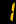# HKDSE

This course caters for students with various backgrounds and abilities. It aims at helping students to consolidate what they have learned through basic education, broadening and deepening their learning experiences, as well as further enhancing their positive values and attitudes towards the learning of mathematics. Topics will be taught:

### Number and Algebra Strand

1. Quadratic equations in one unknown
2. Functions and graphs
3. Exponential and logarithmic functions
6. Variations
7. Arithmetic and geometric sequences and their summations
8. Inequalities and linear programming
9. More about graphs of functions

### Measures, Shape and Space Strand

1. Basic properties of circles
2. Locus
3. Equations of straight lines and circles

### Data Handling Strand

1. Permutation and combination
3. Measures of dispersion
4. Uses and abuses of statistics
5. Further applications
6. Inquiry and investigation

### Learning Objectives

#### Number and Algebra Strand

• extend the concepts of numbers to complex numbers;
• investigate and describe relationships between quantities using algebraic symbols;
• generalize and describe patterns in sequences of numbers using algebraic symbols, and apply the results to solve problems;
• interpret more complex algebraic relations from numerical, symbolic and graphical perspectives;
• manipulate more complex algebraic expressions and relations, and apply the knowledge and skills to formulate and solve real-life problems and justify the validity of the results obtained; and apply the knowledge and skills in the Number and Algebra strand to generalise, describe and communicate mathematical ideas and solve further problems in other strands.

#### Measures, Shape and Space Strand

• use inductive and deductive approaches to study the properties of 2-dimensional shapes;
• formulate and write geometric proofs involving 2-dimensional shapes with appropriate symbols, terminology and reasons;
• inquire into and describe geometric knowledge in 2-dimensional space using algebraic relations and apply this knowledge in solving related problems;
• inquire and describe geometric knowledge in 2-dimensional space and 3-dimensional space using trigonometric functions and apply the knowledge in solving related problems; and
• interconnect the knowledge and skills in the Measures, Shape and Space strand and other strands, and apply them to formulate and solve 2-dimensional and 3-dimensional problems using various strategies.

#### Data Handling Strand

• understand the measures of dispersion;
• select and use the measures of central tendency and dispersion to compare data sets;
• investigate and judge the validity of arguments derived from data sets;
• acquire basic techniques in counting;
• formulate and solve further probability problems by applying simple laws; and
• integrate the knowledge in statistics and probability to solve real-life problems.